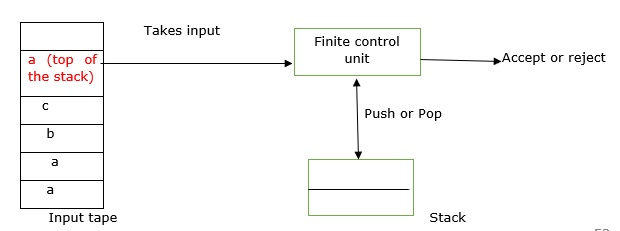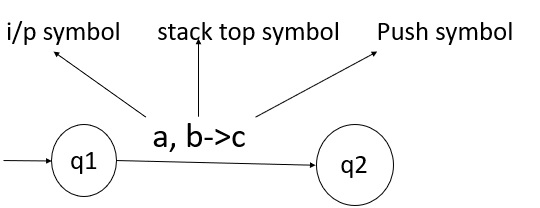# What is Push down automata in TOC?

A push down automata (PDA) is a way to implement a context free grammar (CFG) in a similar way to design the deterministic finite automata (DFA) for a regular grammar.

A DFA can remember a finite amount of information but a PDA can remember an infinite amount of information.

Basically, a PDA is as follows −

"Finite state machine+ a stack"

PDA has three components, which are as follows −

• An input tape.
• A control unit.
• A stack with infinite size.

A PDA may or may not read input symbols, but it has to read the top of the stack in every transaction.A PDA can be formally described as 7-tuples

(Q, ∑,S, δ,q0,I,F)

• Q is a finite number of states.
• ∑ is the input alphabet.
• S is a stack symbol.
• δ is the transition function: QX(∑U{e})XSXQ
• q0 is the initial state (q0 belongs to Q).
• I is the initial state top symbol.
• F is a set of accepting states (F belongs to Q).

The diagram shows a transition in PDA from a state q1 to state q2 labeled as a,b->cAt state q1, if an input string 'a' is encountered and the top symbol of stack is 'b' then we pop 'b', push 'c' on top of the stack and move to state q2.